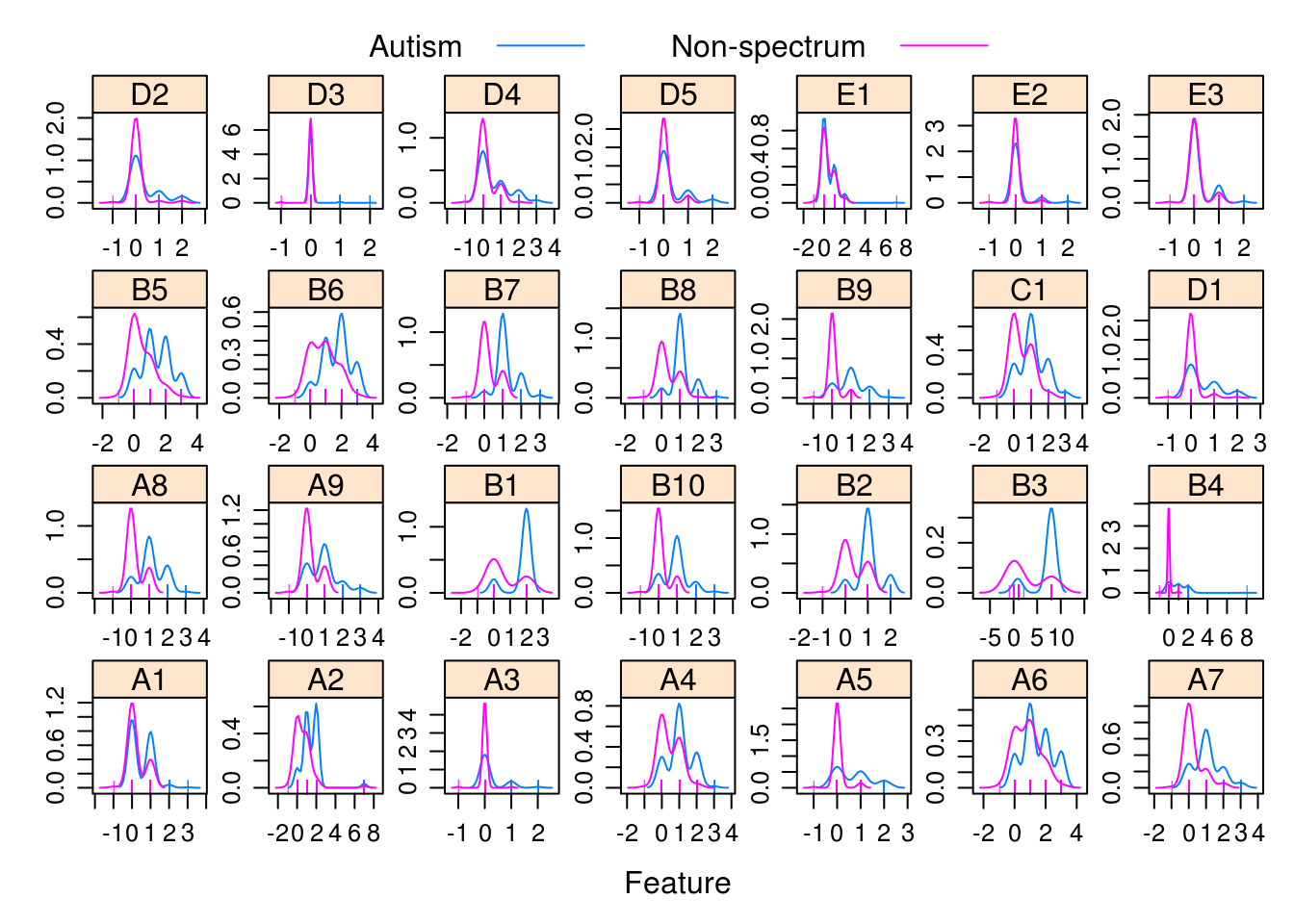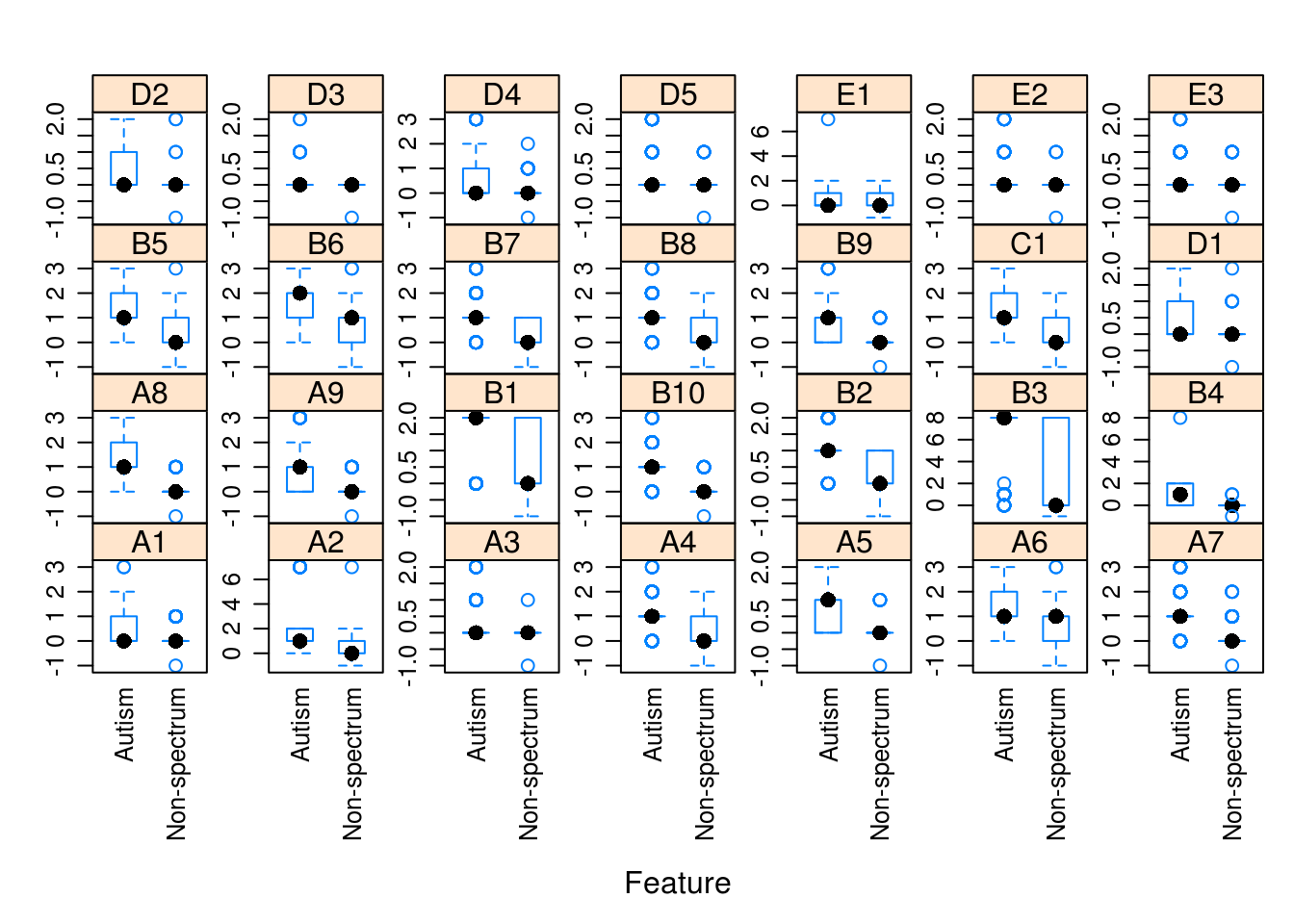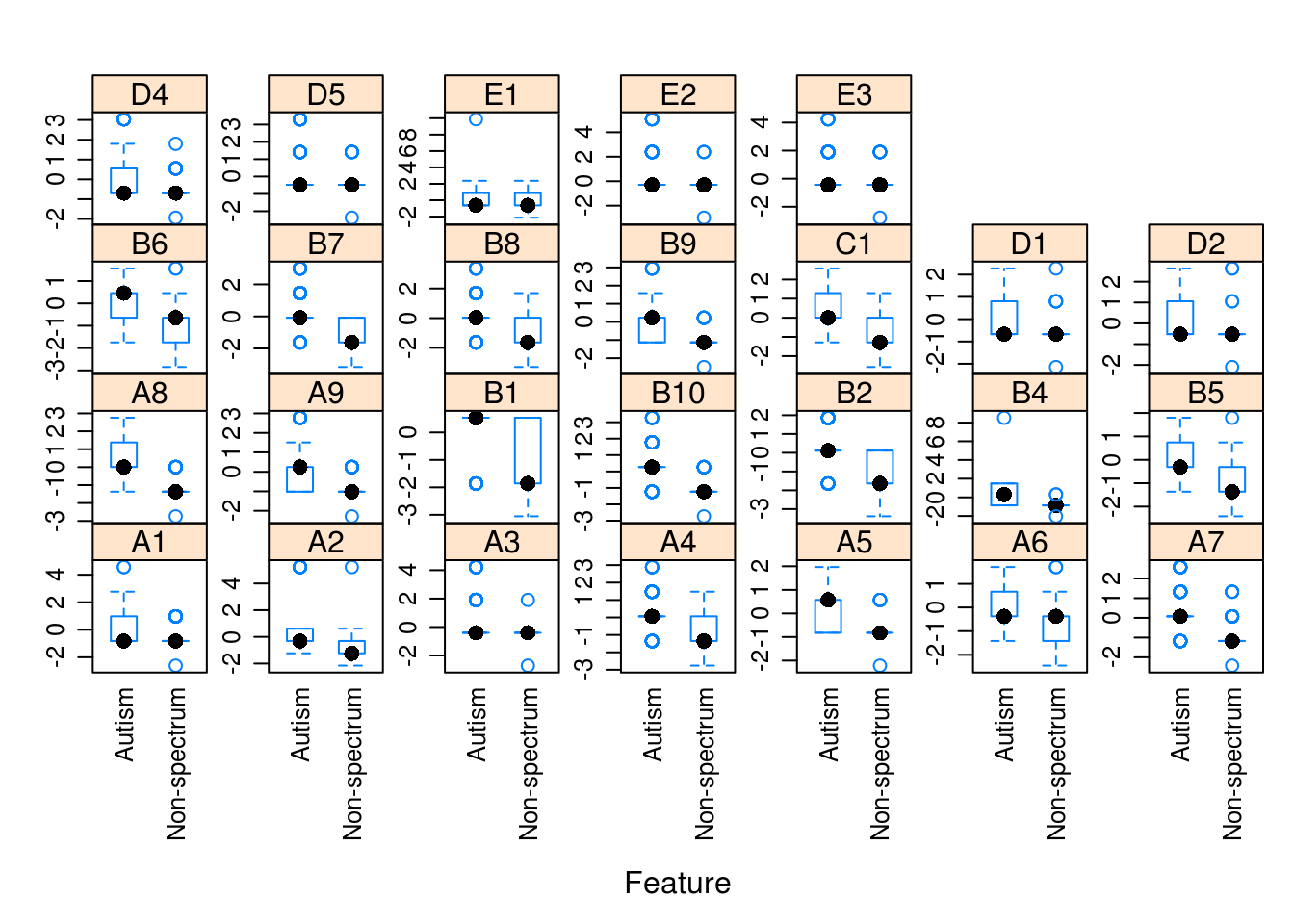This tutorial will walk through data visualization, model building (training), feature selection, and finally, testing using a machine learning package called “caret” that serves as a wrapper for pretty much any algorithm that you can think of.

``library(caret)``
``````## Loading required package: lattice

First let’s read in our data. These are ADOS2 Module 3 questions to predict autism diagnosis.

## Testing Data

``````setwd("/home/vanessa/Documents/Dropbox/Research/Papers/MachineLearning_Weka/ADOS2_m3")

## Training data

``````setwd("/home/vanessa/Documents/Dropbox/Research/Papers/MachineLearning_Weka/ADOS2_m3")

# Here are all the questions
questions = c("A1","A2","A3","A4","A5","A6","A7","A8","A9","B1","B2","B3","B4","B5","B6","B7","B8","B9","B10","C1","D1","D2","D3","D4","D5","E1","E2","E3")
df <- subset(df,select = c(questions,"Class"))

# We need to make labels just autism, non-spectrum
tmp = as.character(df\$Class)
tmp[tmp == "Autism Spectrum"] = "Autism"
df\$Class = as.factor(tmp)``````

# VISUALIZATION

Lets have a little fun with our data before we try anything. This will give us a sense of the distributions before we do anything, and which questions will be useful

``````library(AppliedPredictiveModeling)
featurePlot(x=df[,-29],y=df\$Class,plot="density",
scales = list(x = list(relation="free"),
y = list(relation="free")),
pch = "|",
auto.key = list(columns = 2))````````````featurePlot(x = df[,-29],
y = df\$Class,
plot = "box",
scales = list(y = list(relation="free"),
x = list(rot = 90)),
auto.key = list(columns = 2))            ``````# PREPROCESSING

Do we have any variables with little to no variance

``````nzv = nearZeroVar(df)
hist(df[,23],main="This is the variable with piddley variance",xlab="question response")````````filtered = df[, -nzv]``

Correlated Vars?

``````corrs = cor(filtered[,-28])
highcorr = findCorrelation(corrs, cutoff = 0.75)
filtered = filtered[-highcorr]``````

Linear dependencies? (eg, A1 + A2 = A3)

``combos = findLinearCombos(filtered[,-27])``

Now let’s prepare training data for algorithms, first we should scale to have unit variance, 0 mean

``````preprocess = preProcess(filtered[,-27], method = c("center", "scale"))
training = predict(preprocess, filtered[,-27])``````

Take another look. The awesome thing about these plots is that they make the selected questions SO obvious! This tells us that it doesn’t matter so much what method we use for feature selection - the signal is real in the data and that’s why this classifier works so well.

``````featurePlot(x = training,
y = filtered\$Class,
plot = "box",
scales = list(y = list(relation="free"),
x = list(rot = 90)),
auto.key = list(columns = 2))            ``````# TRAINING

Take a look at all the models we can choose from!

The great thing about caret is that it’s just a wrapper for most Machine Learning libraries in R. We could even write a function to test all of them - but let’s start simple and just do a few.

We already have our training data defined, “training”

``training = cbind(training,Class = df\$Class)``

Let’s first set up the kind of resampling we want to do. There is bootstrap, LOOCV, CV

Here is a function to calculate sensitivity, specificity, AUC

``head(twoClassSummary)``
``````##
## 1 function (data, lev = NULL, model = NULL)
## 2 {
## 3     require(pROC)
## 4     if (!all(levels(data[, "pred"]) == levels(data[, "obs"])))
## 5         stop("levels of observed and predicted data do not match")
## 6     rocObject <- try(pROC::roc(data\$obs, data[, lev]), silent = TRUE)``````

We can give this function to “trainControl” to tell it to give us those accuracy metrics.

Here is 10 fold CV, repeated 10 times. The default are just accuracy and Kappa, but we’ve changed it to output ROC metrics. This function uses trapezoidal rule to calculate accuracy

``````fitControl <- trainControl(method = "repeatedcv",number = 10,
repeats = 10,
classProbs = TRUE,
summaryFunction = twoClassSummary)
# methods can be "boot", "cv", "LOOCV", "LGOCV", "repeatedcv", "timeslice", "none" and "oob".
# number is number of folds in CV``````

If you want just accuracy, change summaryFunction to defaultSummary, and specify “Accuracy” in metric below. Or just specify nothing, because the default for classification models is to use accuracy and Kappa for validation.

Now let’s train our data - stochastic gradient boosting. Just a heads up - this is going to spit out a warning about the class labels (having a “-” that is converted to “.”). It should be fixed when you do this for realsies, but it doesn’t negatively impact us because we aren’t calculating probabilities.

``````# install.packages('pROC')
fit.gbm <- train(Class ~ ., data = training,
method = "gbm",
trControl = fitControl,
verbose = FALSE,
metric="ROC")``````
``````## Loading required package: gbm
##
## Attaching package: 'survival'
##
## The following object is masked from 'package:caret':
##
##     cluster
##
``````## Warning: At least one of the class levels are not valid R variables names;
## This may cause errors if class probabilities are generated because the
## variables names will be converted to: Autism, Non.spectrum``````
``````## Loading required package: pROC
## Type 'citation("pROC")' for a citation.
##
## Attaching package: 'pROC'
##
## The following objects are masked from 'package:stats':
##
##     cov, smooth, var``````

Caret makes it easy to plot how parameter selection influences accuracy.

``````trellis.par.set(caretTheme())
ggplot(fit.gbm)``````